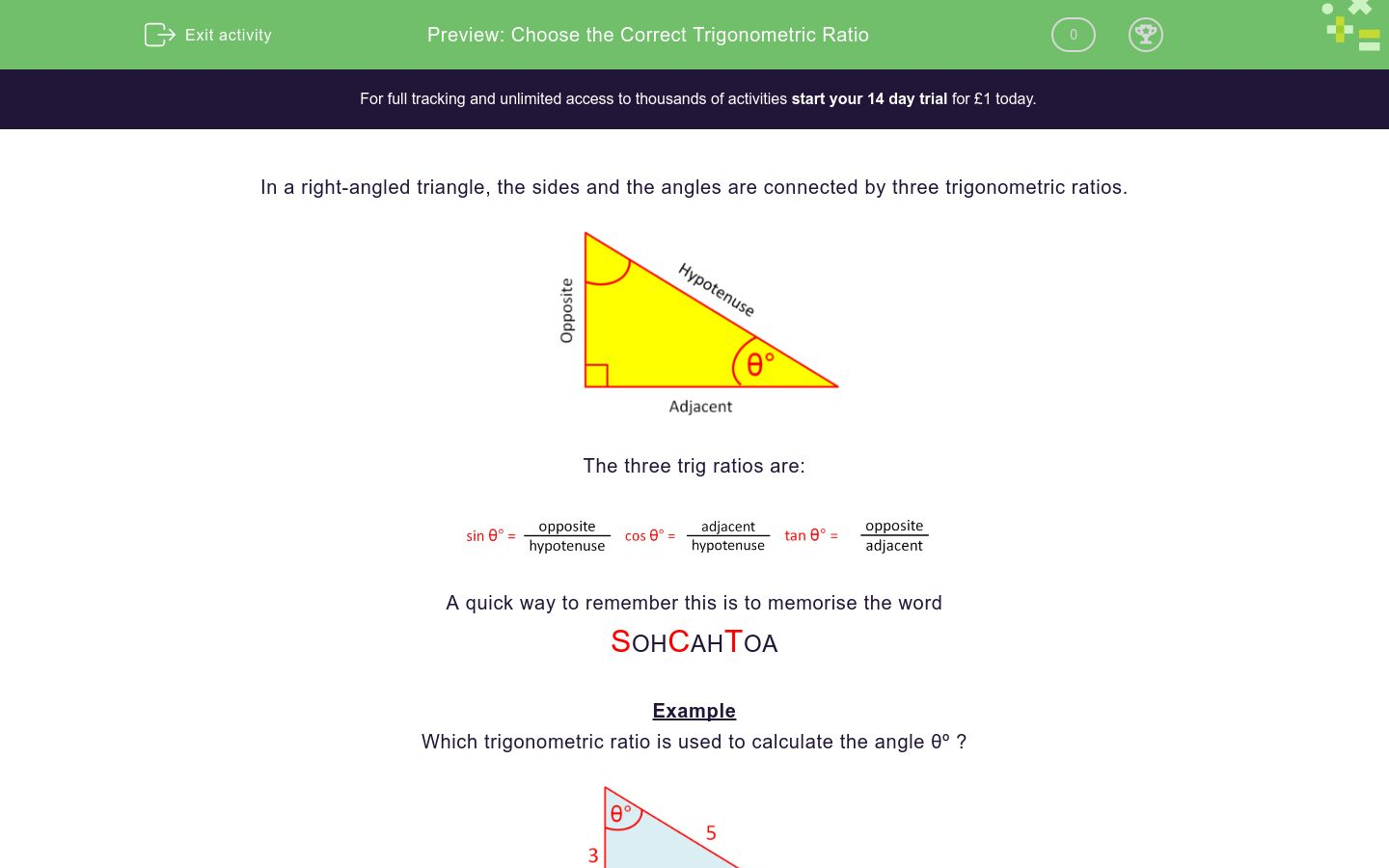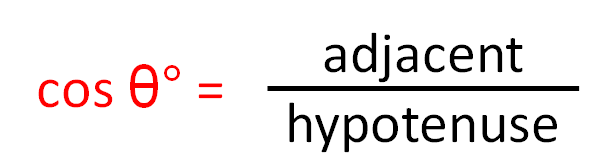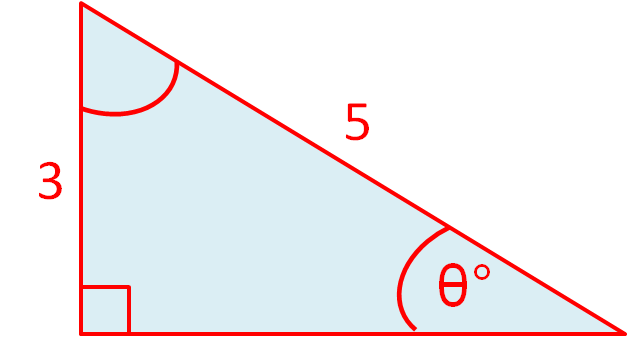# Choose the Correct Trigonometric Ratio

In this worksheet, students select the correct trigonometric ratio to find the given angle.Key stage:  KS 3

Curriculum topic:   Geometry and Measures

Curriculum subtopic:   Solve Right-Angled Triangle Problems

Difficulty level:### QUESTION 1 of 10

In a right-angled triangle, the sides and the angles are connected by three trigonometric ratios.The three trig ratios are:A quick way to remember this is to memorise the word

SOHCAHTOA

Example

Which trigonometric ratio is used to calculate the angle θº ?Label the sides adjacent, opposite and hypotenuse in relation to the angle θº.

The given sides are the adjacent and the hypotenuse.SOHCAHTOA

The correct trig ratio is COS.

Which trigonometric ratio is used to calculate the angle θº ?sin

cos

tan

Which trigonometric ratio is used to calculate the angle θº ?sin

cos

tan

Which trigonometric ratio is used to calculate the angle θº ?sin

cos

tan

Which trigonometric ratio is used to calculate the angle θº ?sin

cos

tan

Which trigonometric ratio is used to calculate the angle θº ?sin

cos

tan

Which trigonometric ratio is used to calculate the angle θº ?sin

cos

tan

Which trigonometric ratio is used to calculate the angle θº ?sin

cos

tan

Which trigonometric ratio is used to calculate the angle θº ?sin

cos

tan

Which trigonometric ratio is used to calculate the angle θº ?sin

cos

tan

Which trigonometric ratio is used to calculate the angle θº ?sin

cos

tan

• Question 1

Which trigonometric ratio is used to calculate the angle θº ?sin
• Question 2

Which trigonometric ratio is used to calculate the angle θº ?tan
• Question 3

Which trigonometric ratio is used to calculate the angle θº ?tan
• Question 4

Which trigonometric ratio is used to calculate the angle θº ?sin
• Question 5

Which trigonometric ratio is used to calculate the angle θº ?cos
• Question 6

Which trigonometric ratio is used to calculate the angle θº ?tan
• Question 7

Which trigonometric ratio is used to calculate the angle θº ?sin
• Question 8

Which trigonometric ratio is used to calculate the angle θº ?tan
• Question 9

Which trigonometric ratio is used to calculate the angle θº ?sin
• Question 10

Which trigonometric ratio is used to calculate the angle θº ?cos
---- OR ----

Sign up for a £1 trial so you can track and measure your child's progress on this activity.

### What is EdPlace?

We're your National Curriculum aligned online education content provider helping each child succeed in English, maths and science from year 1 to GCSE. With an EdPlace account you’ll be able to track and measure progress, helping each child achieve their best. We build confidence and attainment by personalising each child’s learning at a level that suits them.

Get started